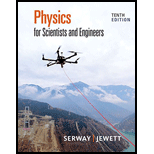Chapter 31, Problem 13PPhysics for Scientists and Enginee...

10th Edition
Raymond A. Serway + 1 other
ISBN: 9781337553278

Solutions

Chapter
SectionPhysics for Scientists and Enginee...

10th Edition
Raymond A. Serway + 1 other
ISBN: 9781337553278
Textbook Problem

A circuit consists of a coil, a switch, and a battery, all in series. The internal resistance of the battery is negligible compared with that of the coil. The switch is originally open. It is thrown closed, and after a time interval Δt, the current in the circuit reaches 80.0% of its final value. The switch then remains closed for a time interval much longer than Δt. The wires connected to the terminals of the battery are then short-circuited with another wire and removed from the battery, so that the current is uninterrupted. (a) At an instant that is a time interval Δt after the short circuit, the current is what percentage of its maximum value? (b) At the moment 2Δt after the coil is short-circuited, the current in the coil is what percentage of its maximum value?

(a)

To determine
The percentage of current to its maximum value at an instant that is time interval Δt after short circuit.

Explanation

Given info: the percentage of current to its final value is 80.0% and time interval after short circuit is Δt .

Explanation:

Formula to calculate current at any time Δt is,

i=Imax(1eRLΔt) (1)

Here,

Imax is the maximum current in circuit.

R is resistance in circuit.

L is inductance in circuit.

As per given condition,

i=0.8Imax

Substitute 0.8Imax for i in equation (I).

0.8Imax=Imax(1eRLΔt)0.8=(1eRLΔt)eRLΔt=10

(b)

To determine
The percentage of current to its maximum value at an instant that is time interval 2 Δt after short circuit

Still sussing out bartleby?

Check out a sample textbook solution.

See a sample solution

The Solution to Your Study Problems

Bartleby provides explanations to thousands of textbook problems written by our experts, many with advanced degrees!

Get Started

Find more solutions based on key concepts